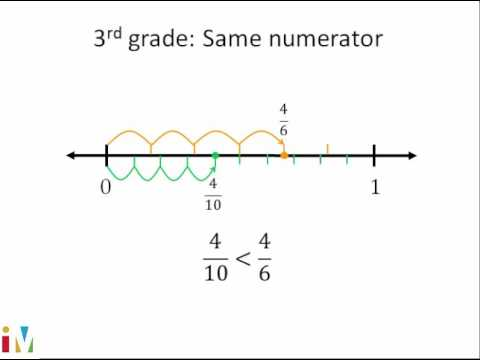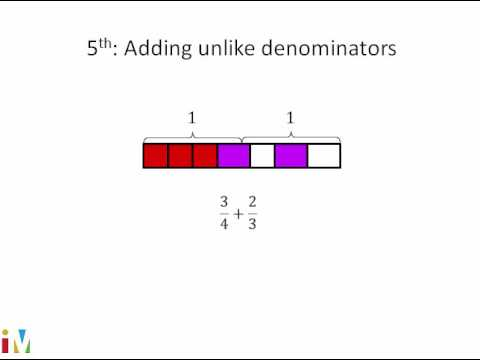Video

# Visually dividing unit fraction by a whole number (Full video)

Description: This video uses an area model to explain 1/7 ÷ 4. Each of those is a seventh, and we have one of those sevenths filled in, so this is 1/7 right over here, and then they divide it into four equal sections. In fact, they divide all of the sevenths into four equal sections, and so 1/7, which is this whole green bar divided by four, well what would be this fraction of the whole that is in a question mark. Can you pause this video and figure out what fraction of the whole is this question mark?

### Other videos you might be interested in### Comparing Fractions

#### Illustrative Mathematics